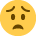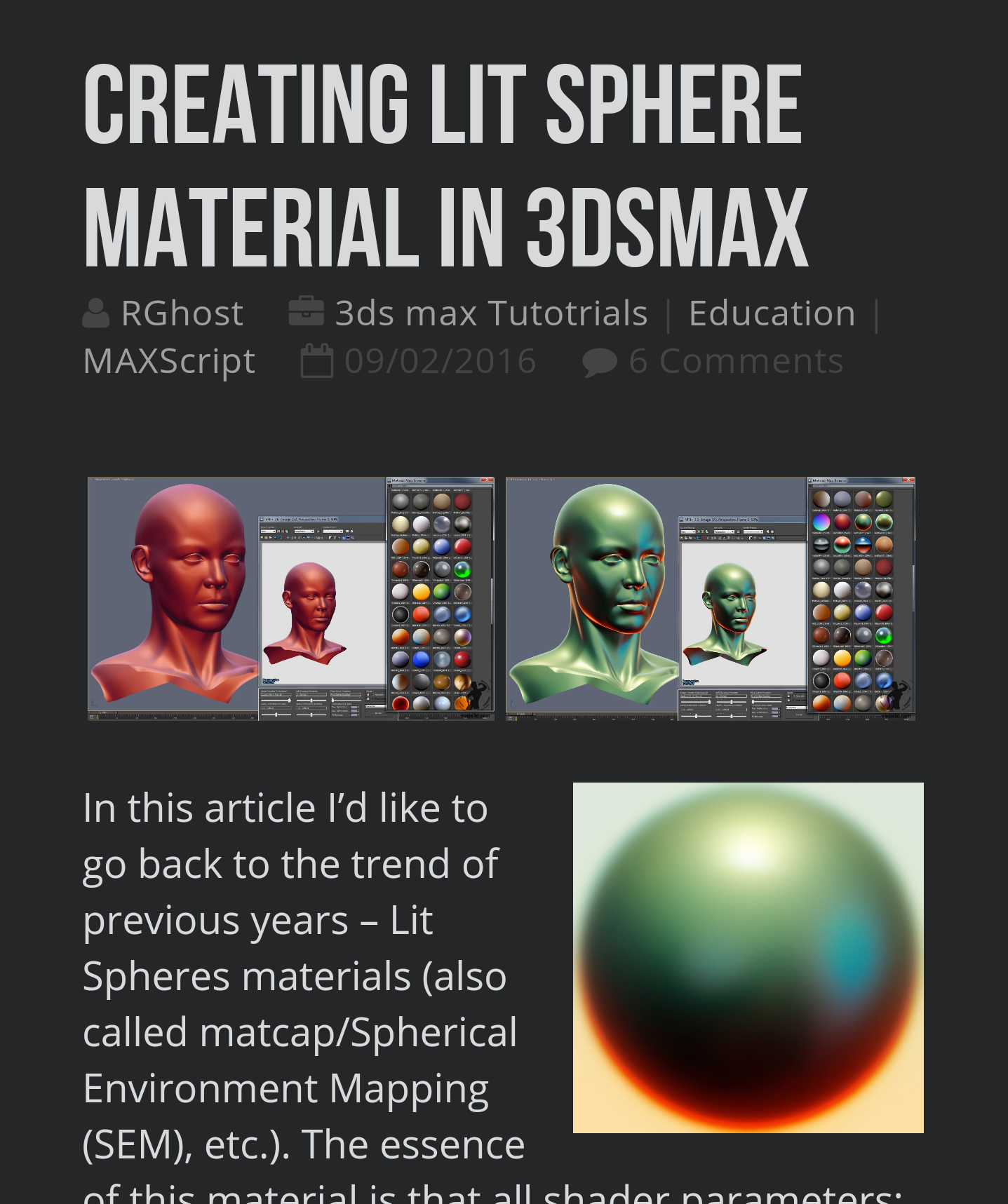# LitSpheres in Max viewport

## Replies

• Offline / Send Message
polycounter lvl 7
Got these badboys working in UDK over here incase anyone is curious Including a hacked world space option (instead of camera space).

Thanks for all the info here, couldn't have done it without it.
• Offline / Send Message
I couldn't find an HLSL matcap shader that worked with XNA so I built this from the previous Unity one.

```#define MaxBones 59
float4x4 Bones[MaxBones];
float4x4 View;
float4x4 Projection;
float4x4 WorldInverseTranspose;
float4x4 World;

float4 MainColor = float4(1,1,1,1);
float3 lightDir1 = float3(1,1,1);
float3 lightDir2 = float3(-1,-1,-1);
float4 specularPower = 30;
float Kd = 1;
float Ks = 1;
float Kr = 1;

bool useTextureMap = false;
bool useSpecMap = true;
bool useNormalMap = false;
bool useEnvMap = false;
bool useMatcap = false;

//Texture Map
texture ColorMap;
sampler C_Sampler = sampler_state {
Texture = <ColorMap>;
MinFilter = Linear;
MagFilter = Linear;
MipFilter = Linear;
};

//Normal Map
texture NormalMap;
sampler N_Sampler = sampler_state {
Texture = <NormalMap>;
MinFilter = Linear;
MagFilter = Linear;
MipFilter = Linear;
};

//Environment Map
texture EnvironmentMap;
sampler Env_Sampler = sampler_state{
Texture = <EnvironmentMap>;
MinFilter = Linear;
MagFilter = Linear;
MipFilter = Linear;

};

//Specular Map
texture SpecularMap;
sampler Spec_Sampler = sampler_state{
Texture = <SpecularMap>;
MinFilter = Linear;
MagFilter = Linear;
MipFilter = Linear;
};

//Matcap Map
texture MatcapMap;
sampler Matcap_Sampler = sampler_state{
Texture = <MatcapMap>;
MinFilter = Linear;
MagFilter = Linear;
MipFilter = Linear;
};

struct VS_INPUT
{
float4 Position : POSITION0;
float2 TexCoord : TEXCOORD0; //Gets and stores the UV information
float3 Normal : NORMAL0;
float3 Tangent : TANGENT0;
float4 BoneIndices : BLENDINDICES0;
float4 BoneWeights : BLENDWEIGHT0;

float3  TtoV0 : TEXCOORD1;
float3  TtoV1 : TEXCOORD2;
};

struct VS_OUTPUT
{
float4 Position : POSITION0;
float2 TexCoord : TEXCOORD0; //UV coord output
float3 Data1 : TEXCOORD1;
float3 Data2 : TEXCOORD2;
float3 Data3 : TEXCOORD3;
float3 Tangent : TEXCOORD6;
float3  TtoV0 : TEXCOORD4;
float3  TtoV1 : TEXCOORD5;
};

VS_OUTPUT VSBasic(VS_INPUT input) {
VS_OUTPUT output;

//For every bone transform
float4x4 skinTransform = 0;
skinTransform += Bones[input.BoneIndices.x] * input.BoneWeights.x;
skinTransform += Bones[input.BoneIndices.y] * input.BoneWeights.y;
skinTransform += Bones[input.BoneIndices.z] * input.BoneWeights.z;
skinTransform += Bones[input.BoneIndices.w] * input.BoneWeights.w;

//Transforming the point with the bone transforms, then view matrix and projection matrix
float4 pos = mul(input.Position, skinTransform);
float3 eyeLoc = mul(View._m30_m31_m32, transpose(View));
float3 eyeDir = eyeLoc - pos;

pos = mul(pos,View);
pos = mul(pos,Projection);

//The normals
float3 nml = mul(input.Normal, skinTransform);
nml = normalize(nml); //Normalize the normal

output.Position = pos;
output.TexCoord = input.TexCoord;

output.Data1 = nml;
output.Data2 = eyeDir;
output.Data3 = 0;
return output;
}

VS_OUTPUT VSNormals(VS_INPUT input) {
VS_OUTPUT output;

//For every bone transform
float4x4 skinTransform = 0;
skinTransform += Bones[input.BoneIndices.x] * input.BoneWeights.x;
skinTransform += Bones[input.BoneIndices.y] * input.BoneWeights.y;
skinTransform += Bones[input.BoneIndices.z] * input.BoneWeights.z;
skinTransform += Bones[input.BoneIndices.w] * input.BoneWeights.w;

//Transforming the point with the bone transforms, then view matrix and projection matrix
float4 pos = mul(input.Position, skinTransform);
float3 eyeLoc = mul(View._m30_m31_m32, transpose(View));
float3 eyeDir = eyeLoc - pos;

pos = mul(pos,View);
pos = mul(pos,Projection);

//Tangent, normal, binormal
float3x3 tangentSpace;
tangentSpace = mul(input.Tangent, skinTransform);
tangentSpace = mul(cross(input.Tangent, input.Normal), skinTransform);
tangentSpace = mul(input.Normal, skinTransform);

float3 tgtLightDir1 = mul(tangentSpace, lightDir1);
float3 tgtLightDir2 = mul(tangentSpace, lightDir2);

output.Position = pos;
output.TexCoord = input.TexCoord;

output.Data1 = normalize(tgtLightDir1);
output.Data2 = normalize(tgtLightDir2);
output.Data3 = normalize(mul(tangentSpace,eyeDir));

output.Tangent = input.Tangent;

output.TtoV0 = tgtLightDir1;
output.TtoV1 = tgtLightDir2;

output.TtoV0 = normalize(mul(tangentSpace, WorldInverseTranspose.xyz));
output.TtoV1 = normalize(mul(tangentSpace, WorldInverseTranspose.xyz));

return output;
}

float4 PSNormals(VS_OUTPUT input) : COLOR0{

float4 outColor = tex2D(C_Sampler, input.Tangent);
float3 normal =  2 * tex2D(N_Sampler, input.Tangent)- 1.0;
normal = normalize(normal);

half2 vn;
vn.x = dot(input.TtoV0, normal);
vn.y = dot(input.TtoV1, normal);

float4 matcapLookup = tex2D(Matcap_Sampler, vn*0.5 + 0.5);

if (useTextureMap){
matcapLookup.a = tex2D(C_Sampler, input.Tangent).a*0.5;
matcapLookup.rgb += tex2D(C_Sampler, input.Tangent).rgb*0.5-0.4;
}

return matcapLookup;
}

technique Technique1
{
pass Pass1
{

ZEnable = true;
//CullMode = CCW;

}
}
```
• Offline / Send Message
This thread deserves a necro. Great shader for previewing surfaces and smoothing.

It works fine for me with nitrous in 2012, but unfortunately it doesn't work with SSAO.
I'd pay for a matcap shader with working SSAO• Offline / Send Message
polycounter lvl 11
I fixed Matballz shader to get it work with directx11 and wrote maxscript for creating material library with matcaps. http://veda3d.com/creating-lit-sphere-material-in-3dsmax/• Offline / Send Message
high dynamic range
RGhost said:
I fixed Matballz shader to get it work with directx11 and wrote maxscript for creating material library with matcaps. http://veda3d.com/creating-lit-sphere-material-in-3dsmax/

Thanks for your article. I renewed my interest in this a little while ago and came across your post. I didn't need to look any further, so thanks. I made a quick vid tut a while back, referencing your site and information. Hope you don't mind.

• Offline / Send Message
polycounter lvl 11
RGhost said:
I fixed Matballz shader to get it work with directx11 and wrote maxscript for creating material library with matcaps. http://veda3d.com/creating-lit-sphere-material-in-3dsmax/

Thanks for your article. I renewed my interest in this a little while ago and came across your post. I didn't need to look any further, so thanks. I made a quick vid tut a while back, referencing your site and information. Hope you don't mind.
Glad that you find this article useful.I like your vid, I will add link to it  in my article.
• Offline / Send Message
polycounter lvl 8
Could someone please post the original fx version that worked with DirectX 9 ? The link at the beginning of this threat or on veda3D is dead. And I really need (for plugins reasons) to stick with 3DSMax 2013 which doesn't have DirectX11 available in the viewports Display Drivers !
Hope someone still have the file ! (Matballz.fx )
• Offline / Send Message
polycounter lvl 11
Could someone please post the original fx version that worked with DirectX 9 ? The link at the beginning of this threat or on veda3D is dead. And I really need (for plugins reasons) to stick with 3DSMax 2013 which doesn't have DirectX11 available in the viewports Display Drivers !
Hope someone still have the file ! (Matballz.fx )